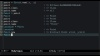In Progress
Unit 1, Lesson 1
In Progress

# StructVideo transcript & code

I've used Structs in passing in several episodes already, so I figured it was about time I did a proper introduction to `Struct`.

Here's a typical Ruby class definition for a `Point` class. The class has two attributes, `x` and `y`, and an initializer with positional arguments for setting the two attributes.

``````class Point
attr_accessor :x
attr_accessor :y

def initialize(x=nil,y=nil)
@x = x
@y = y
end
end
``````

While fairly concise by many language' standards, this definition still seems a little redundant. The tokens `x` and `y` are each referenced a total of four times. It would be nice if we could eliminate some of the duplication in this very common case.

Ruby has a tool to help, and its name is `Struct`. Let's create a `Struct` with `x` and `y` coordinates.

``````point = Struct.new(:x, :y)
``````

Just what exactly have we created here? Let's take a closer look.

``````point = Struct.new(:x, :y)
point # => #<Class:0x00000004da22e0>
point.class # => Class
point.name  # => nil

``````

So we called `.new` on a class and… we got another class! And this new class has no name.

Typically when we create Structs in Ruby we immediately assign the resulting class to a constant. Let's assign our `Struct` to a constant called `Point`.

``````Point = point
Point # => Point
point # => Point
point.class # => Class
point.name  # => "Point"
``````

Looking at this, you might be suspecting some slight of hand. Before we assigned the Struct to the `Point` constant, it had no name. But afterward, it has the name "Point" - even when we reference it through the original local variable that we assigned it to.

It turns out that Ruby has a very special rule for when an anonymous class or module is assigned to a constant for the first time. When that happens, Ruby sets the name of the class or module to the name of the constant. This only happens once:

``````Point = Struct.new(:x, :y)
Point                           # => Point
Location = Point
Location                        # => Point
``````

This is the only time that assigning an object to a variable or constant causes a change to the object itself.

So now that we have a `Point` class generated by `Struct`, what can we do with it?

Well, we can instantiate `Point` objects:

``````Point = Struct.new(:x, :y)
Point.new                       # => #<struct Point x=nil, y=nil>
Point.new(23)                   # => #<struct Point x=23, y=nil>
Point.new(5,7)                  # => #<struct Point x=5, y=7>

``````

We can also get and set the `x` and `y` attribute values.

``````p = Point.new(4,5)
p.x                             # => 4
p.y                             # => 5
p.x = 7
p.x                             # => 7
``````

So we can see that so far, this one-line `Struct` is equivalent to the 8-line class we started out with. But `Struct` doesn't stop there.

We can also get and set values using Hash-like subscript syntax. Symbols and strings can be used interchangeably as keys.

``````p = Point.new(4,5)
p[:x]                           # => 4
p["y"]                          # => 5
p[:x] = 13
p.x                             # => 13
``````

We also get the equality operator for free. `Struct` defines it so that instances with equal attributes are considered equal.

``````Point = Struct.new(:x, :y)
Point.new(5,3) == Point.new(5,3) # => true
Point.new(5,3) == Point.new(3,5) # => false
``````

But it doesn't stop there. Unlike attributes defined with `attr_accessor`, structs can introspect and iterate over their attributes. We can ask a point instance for the names of its attributes with `#members`, iterate over the values with `#each`, or iterate over names and values with `each_pair`.

``````p = Point.new(3,5)
p.members                       # => [:x, :y]
p.each do |value|
puts value
end
p.each_pair do |name, value|
puts "#{name}: #{value}"
end
# >> 3
# >> 5
# >> x: 3
# >> y: 5
``````

To top it off, structs include `Enumerable`, so we have the full complement of `Enumerable` methods as well.

``````p = Point.new(3,5)
p.max                           # => 5
p.reduce(&:+)                   # => 8
``````

But what if we want more than just attribute-related methods? Do we have to revert to a traditional class definition?

No we don't! The Struct constructor can also take a block. Inside the block we can define our own methods, just as we would in a class definition. Here's a custom `#to_s` method for our `Point` class.

``````Point = Struct.new(:x, :y) do
def to_s
"(#{x}x#{y})"
end
end
Point.new(3,5).to_s             # => "(3x5)"
``````

In conclusion: `Struct` is awesome. It's a big timesaver, and a powerful tool for defining rich data structures. If you aren't using it already, I highly recommend taking some time to play around and get familiar with Struct's capabilities.

Happy hacking!